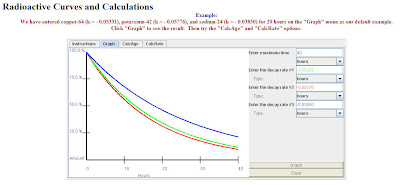This Radioactive Decay Java applet can be found in the National Curve Bank. It was written by Cindy So (Cal State LA) and includes all the data you’d ever need to do realistic radioactive decay problems.You can use the Java applet to compare graphs of elements with different decay rates, and calculate the age of an item based on the original and remaining amounts, among other things.

There is also a nice historical plot of the Carbon-14 decay rate with various items and their Carbon-14 dated age.Although radioactie decay problems are often thought of as precalculus level problems, remember that calculus students can also do these problems by looking at the differential equation that describes the rate of decay (also described on this site).

Possibly Related Posts: